Pruning Criteria

Subsections:

Pruning criteria are similar to growth criteria, except that they use the global change of a metric instead of the per-leaf change. In addition, partition is present, pruning statistics are calculated from the validation partition if one is present.

Decision Tree Entropy Pruning Criterion

When you prune by entropy, the entropy is calculated as though the entire data set were a single leaf that is partitioned into the final number of leaves. Thus, it can be expected that the path taken during pruning might not correspond to the reverse of the path taken during growth, even if the pruning and growth metrics are identical.

The change is then based on the global entropy, comparing the entropy when node is preserved to the entropy when the node is pruned back to a leaf.

Decision Tree Gini Pruning Criterion

As with entropy, the change in Gini statistic is calculated based on the change in the global Gini statistic. The equations for this criterion are otherwise identical to the equations shown in the section Gini Splitting Criterion.

Decision Tree Misclassification Rate Pruning Criterion

The misclassification rate (MISC) is simply the number of mispredictions divided by the number of predictions. Thus, for a leaf that has a predicted target level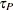, the misclassification rate is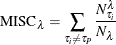For all the leaves in the tree, it is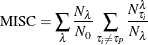The predicted target level is always based on the training data set.

Decision Tree Average Square Error Pruning Criterion

The average square error (ASE) is based on the sum of squares error (SSE). For a perfect assignment, you would expect that the proportion of observations at a leafwould be 1 for the predicted target level and 0 for the remainder. Thus, for a single leaf, the equation for the average of this error is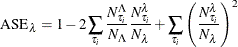whereis for a leaf in the training set and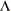is for a leaf in the validation set. If there is no validation set, the training set is used.

Thus, for an ensemble of leaves, the ASE becomes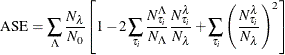This summation is over the validation counts set at the leaves,.

Regression Tree Average Square Error Pruning Criterion

Because the predicted value at each leaf is the average at that leaf, the average square error for a regression tree is simply the standard deviation. Thus, for an ensemble of leaves, the ASE becomes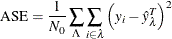where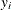is the target value at observationand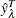is the predicted value at leaf(that is, the average of the target within the training set at that leaf). The T is present to emphasize that it always comes from the training set. This summation is over the validation counts set at the leaves () and over the observations i in the leaf.